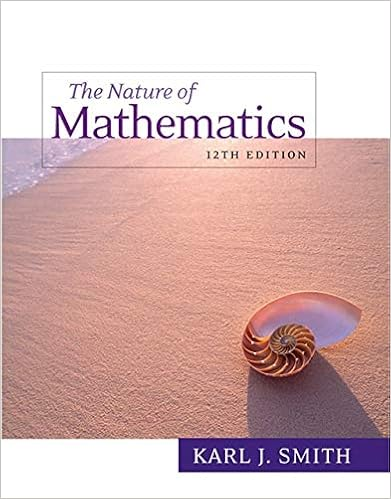# A b c a b a c a b a c multiplyinga b c factoring

• Notes
• 16

This preview shows page 2 - 5 out of 16 pages.

##### We have textbook solutions for you!
The document you are viewing contains questions related to this textbook.The document you are viewing contains questions related to this textbook.
Chapter 5 / Exercise 37
Nature of Mathematics
SmithExpert Verified
a ( b + c ) a b + a c == a b + a c Multiplyinga ( b + c ) Factoring Consider factoring the result of the opening example: 12 x 2 y 3 + 6 xy 2 === 6 xy 2 2 xy + 6 xy 2 1 Factoring 6 xy 2 ( ? ) 6 xy 2 (2 xy +1) We see that the distributive property allows us to write the polynomial 12 x 2 y 3 + 6 xy 2 as a product of the two factors 6 xy 2 and (2 xy +1). Note that in this case, 6 x 2 y is the GCF of the terms of the polynomial. GCF(12 x 2 y 3 , 6 xy 2 )=6 xy 2
##### We have textbook solutions for you!
The document you are viewing contains questions related to this textbook.The document you are viewing contains questions related to this textbook.
Chapter 5 / Exercise 37
Nature of Mathematics
SmithExpert Verified
Factoring out the greatest common factor (GCF)The process of rewriting a polynomial as a product using the GCF of all of its terms. of a polynomial involves rewriting it as a product where a factor is the GCF of all of its terms. 8 x 3 +4 x 2 −16 x 9 ab 2 −18 a 2 b −3 ab == 4 x (2 x 2 + x −4) 3 ab (3 b −6 a −1) ⎫⎭⎬ Fac toring out the GCF To factor out the GCF of a polynomial, we first determine the GCF of all of its terms. Then we can divide each term of the polynomial by this factor as a means to determine the remaining factor after applying the distributive property in reverse. Example 3 Factor out the GCF: 18 x 7 −30 x 5 +6 x 3 . Solution: In this case, the GCF(18, 30, 6) = 6, and the common variable factor with the smallest exponent is x 3 . The GCF of the polynomial is 6 x 3 . 18 x 7 −30 x 5 +6 x 3 = 6 x 3 (?) The missing factor can be found by dividing each term of the original expression by the GCF. 18 x 7 6 x 3 =3 x 4 −30 x 5 6 x 3 =−5 x 2 +6 x 3 6 x 3 =+1 Apply the distributive property (in reverse) using the terms found in the previous step. 18 x 7 −30 x 5 +6 x 3 = 6 x 3 (3 x 4 −5 x 2 +1) If the GCF is the same as one of the terms, then, after the GCF is factored out, a constant term 1 will remain. The importance of remembering the constant term becomes clear when performing the check using the distributive property. 6 x 3 (3 x 4 −5 x 2 +1)== 6 x 3 3 x 4 6 x 3 5 x 2 + 6 x 3 118 x 7 −30 x 5 +6 x 3 Answer: 6 x 3 (3 x 4 −5 x 2 +1) Example 4 Factor out the GCF: 27 x 5 y 5 z +54 x 5 yz −63 x 3 y 4 . Solution: The GCF of the terms is 9 x 3 y . The last term does not have a variable factor of z , and thus z cannot be a part of the greatest common factor. If we divide each term by 9 x 3 y , we obtain 27 x 5 y 5 z 9 x 3 y =3 x 2 y 4 z 54 x 5 yz 9 x 3 y =6 x 2 z −63 x 3 y 4 9 x 3 y =−7 y 3 and can write 27 x 5 y 5 z +54 x 5 yz −63 x 3 y 4 == 9 x 3 y (?)9 x 3 y (3 x 2 y 4 z +6 x 2 z −7 y 3 ) Answer: 9 x 3 y (3 x 2 y 4 z +6 x 2 z −7 y 3 )
Try this! Factor out the GCF: 12 x 3 y 4 −6 x 2 y 3 −3 xy 2 Answer: 3 xy 2 (4 x 2 y 2 −2 xy −1) (click to see video) Of course, not every polynomial with integer coefficients can be factored as a product of polynomials with integer coefficients other than 1 and itself. If this is the case, then we say that it is a prime polynomialA polynomial with integer coefficients that cannot be factored as a product of polynomials with integer coefficients other than 1 and itself.. For example, a linear factor such as 10 x −9 is prime. However, it can be factored as follows: 10 x −9= x (10−9 x )or10 x −9=5(2 x −95) If an x is factored out, the resulting factor is not a polynomial. If any constant is factored out, the resulting polynomial factor will not have integer coefficients. Furthermore, some linear factors
•••